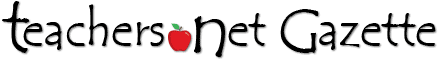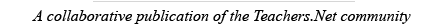SUBSCRIBE | SUBMITDec 2017 Vol 14 No 4BACK ISSUES### Featured Lesson: Multiplying One-Digit NumbersMultiplying One-Digit Numbers is lesson #4749 in the Teachers.Net Lesson Bank

Mathematics, level: elementary
Posted  by Catherine Balmeo
Lincoln Public School, Lincoln, USA
Materials Required: base ten blocks
Activity Time: 30 minutes
Concepts Taught: multiplying numbers using base-ten blocks

Objective:
Apply knowledge of base-ten blocks in order to multiply one-digit numbers

Vocabulary:
factor , product

Materials:
base ten blocks , place value chart,
sentence frame

Engage:
Review regrouping numbers using base-ten blocks. Teacher will distribute base-ten blocks to students.
On the projector, teacher will show 18 ones. Teacher will ask the students to use their blocks to show how to regroup as the teacher models the regrouping. [*This activity will be repeated with other numbers of ones or combinations of tens and ones as the class reviews regrouping numbers.]

Explore:
* What 2 x 13 mean? (2 groups of 13)
*How can we show two 13s with base-ten blocks?
* How can we determine the product of 2 x 13? [Count the blocks]
* What is the product of 2 x 13?

Explanation:
Teacher will show a word problem:
Kawone received 2 boxes of Pokemon cards. Each box holds 32 cards. How many Pokemon cards were in the 2 boxes?

Use base-ten blocks to show 3 groups of 32
* How many tens blocks did you use? [6 tens blocks]
* How many ones blocks did you use [4 ones blocks]
Students will record their answer to the place value chart.
* What is the product of 2 x 32? 
* How many Pokemon cards does Kawone have? 

** [Math problem with regrouping]
Now let’s find the product of 3 x 14
* What does 3 x 14 mean? [3 groups of 14]
* How can we show 3 x 14 with base-ten blocks?
* How many tens blocks did you use? 3 tens blocks
* How many ones blocks did you use? 12 ones blocks
*** Can we trade 10 ones to 1 ten block? Why?
[ 1 ten block is equal to 10 ones ]
* How many tens blocks and ones blocks do you have now? [ 4 tens blocks; 2 ones blocks ]
* What is the product of 3 x 14? 

Elaborate:
•Students will work with their Math Partners. Teacher will distribute the teacher-made worksheet for the partners to complete.
•Teacher will monitor the students as they work
•Each group will be asked to show and explain their work to class.

Evaluate:
The students will work independently to complete the teacher-made formative assessment.

Homework: Students will be given a teacher-made homework for students to practice multiplying one-digit numbers.

Click here to search by keywords for lessons on any subject, or submit your lessons to share!